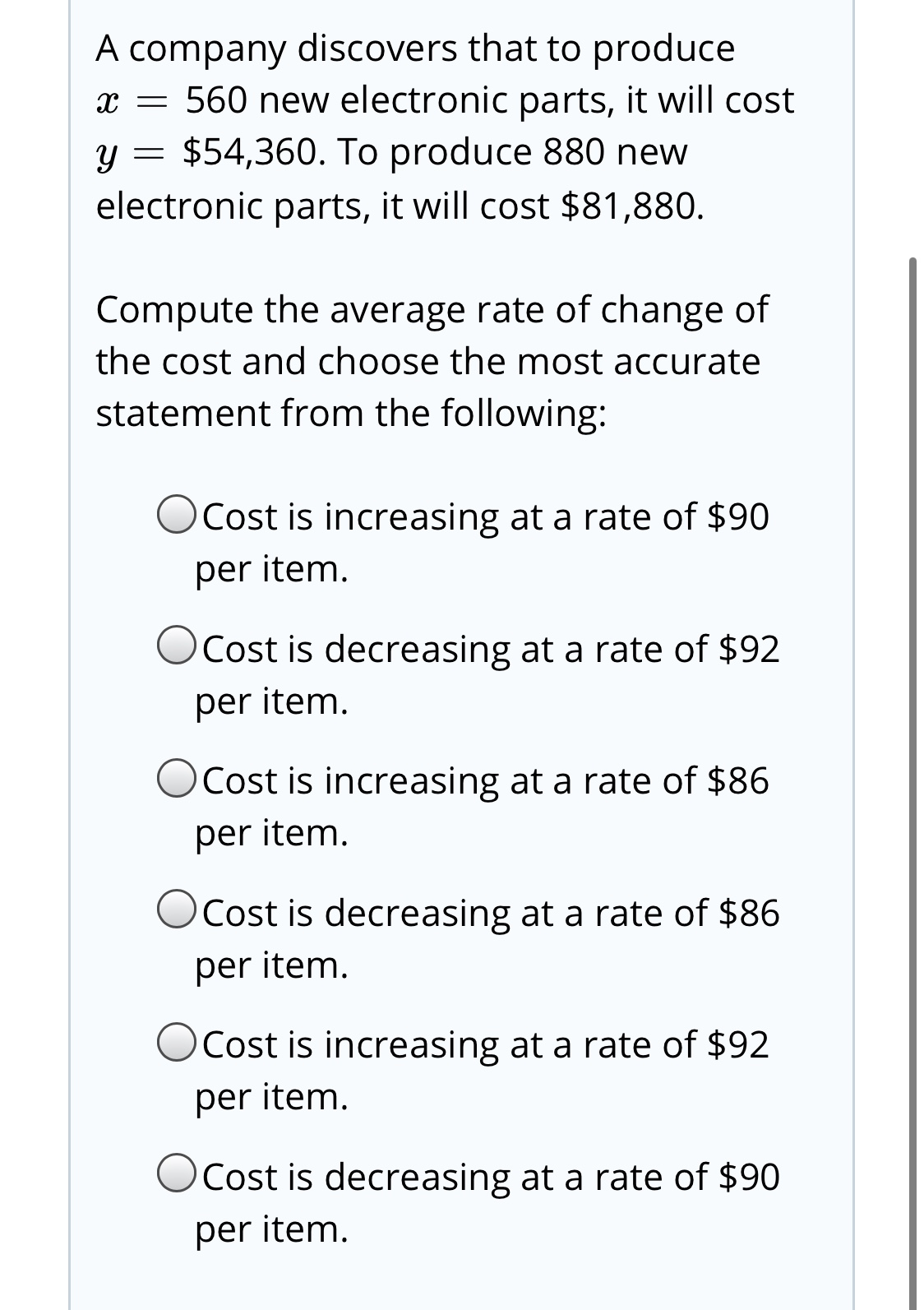# A company discovers that to producex = 560 new electronic parts, it will costy = \$54,360. To produce 880 newelectronic parts, it will cost \$81,880.Compute the average rate of change ofthe cost and choose the most accuratestatement from the following:OCost is increasing at a rate of \$90per item.Cost is decreasing at a rate of \$92per item.OCost is increasing at a rate of \$86per item.OCost is decreasing at a rate of \$86per item.OCost is increasing at a rate of \$92per item.OCost is decreasing at a rate of \$90per item.

Question
115 viewshelp_outlineImage TranscriptioncloseA company discovers that to produce x = 560 new electronic parts, it will cost y = \$54,360. To produce 880 new electronic parts, it will cost \$81,880. Compute the average rate of change of the cost and choose the most accurate statement from the following: OCost is increasing at a rate of \$90 per item. Cost is decreasing at a rate of \$92 per item. OCost is increasing at a rate of \$86 per item. OCost is decreasing at a rate of \$86 per item. OCost is increasing at a rate of \$92 per item. OCost is decreasing at a rate of \$90 per item. fullscreen
check_circle

Step 1

From the given information coordinates are...

### Want to see the full answer?

See Solution

#### Want to see this answer and more?

Solutions are written by subject experts who are available 24/7. Questions are typically answered within 1 hour.*

See Solution
*Response times may vary by subject and question.
Tagged in
MathAlgebra

### Other